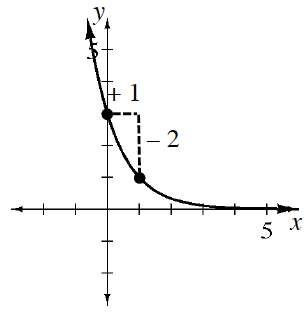### Home > CC3MN > Chapter 10 > Lesson 10.1.2 > Problem10-20

10-20.

Use the graph at right to write an equation in $y = ab^x$ form.

The $a$ value is the initial value or $y$-intercept.

The $b$ value is the multiplier. Think of the two given points in a table like you used in finding geometric sequences. What number would you multiply $3$ by to get $1$?